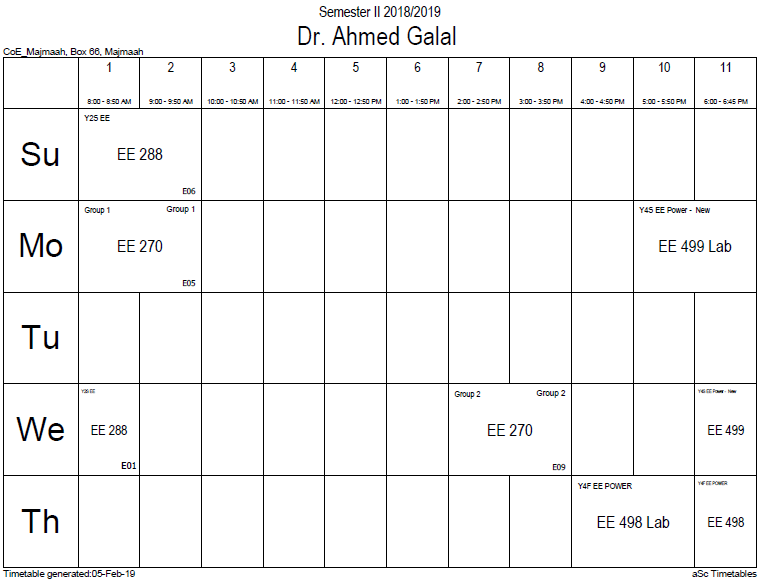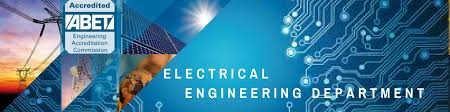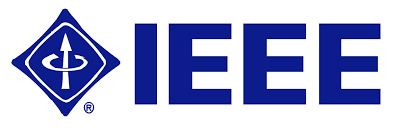## Discrete mathemati

Discrete mathematics is the study of mathematical structures that are fundamentally discrete rather than continuous. In contrast to real numbers that have the property of varying "smoothly", the objects studied in discrete mathematics – such as integers, graphs, and statements in logic – do not vary smoothly in this way, but have distinct, separated values. Discrete mathematics therefore excludes topics in "continuous mathematics" such as calculus and analysis. Discrete objects can often be enumerated by integers. More formally, discrete mathematics has been characterized as the branch of mathematics dealing with countable sets (sets that have the same cardinality as subsets of the natural numbers, including rational numbers but not real numbers). However, there is no exact, universally agreed, definition of the term "discrete mathematics." Indeed, discrete mathematics is described less by what is included than by what is excluded: continuously varying quantities and related notions.

The set of objects studied in discrete mathematics can be finite or infinite. The term finite mathematics is sometimes applied to parts of the field of discrete mathematics that deals with finite sets, particularly those areas relevant to business.

Research in discrete mathematics increased in the latter half of the twentieth century partly due to the development of digital computers which operate in discrete steps and store data in discrete bits. Concepts and notations from discrete mathematics are useful in studying and describing objects and problems in branches of computer science, such as computer algorithms, programming languages, cryptography, automated theorem proving, and software development. Conversely, computer implementations are significant in applying ideas from discrete mathematics to real-world problems, such as in operations research.

Although the main objects of study in discrete mathematics are discrete objects, analytic methods from continuous mathematics are often employed as well.

### Office HoursNo office hours### My Timetable### Contactsemail: [email protected]

Phone: 2570

### Welcome

Welcome To Faculty of Engineering### IEEEhttp://www.ieee.org/

/

### Bookmarkshttp://www.utk.edu/research/

http://science.doe.gov/grants/index.asp

http://www1.eere.energy.gov/vehiclesandfuels/

http://www.eere.energy.gov/

### Upcoming Conferences### Engineering quotes### Travel Web Siteshttp://www.hotels.com/

http://www.orbitz.com/

http://www.hotwire.com/us/index.jsp

http://www.kayak.com/

### Blackboardستقام اختبارات الميدتيرم يوم الثلاثاء 26-6-1440

حسب الجدول المعلن بلوحات الاعلان

### Summer trainingThe registration for summer training will start from 5th week of second semesterClass registration week 1

### برنامج التجسير### إحصائية الموقع

عدد الصفحات: 2879

البحوث والمحاضرات: 1280

الزيارات: 99512